# 4. Verification of Wada Basin Boundaries

In order to more rigorously confirm that the basin boundaries in this three-disk system possess the Wada property, we introduce a surface of section corresponding to the surfaces of the three scattering disks. Since we are only interested in trajectories that enter the scattering region, the position of the particle in the surface of section when it is in the scattering region will be parameterized by the arc length s measured in a counterclockwise direction along the three arcs contained in the scattering region (refer to Fig. 5). Note that in Fig. 5, we have normalized the total arc length to 1, and the point at the end of each arc has the same parameterization as the corresponding point on the disk in the opposing side. To uniquely specify the course of the trajectory, we introduce z = cos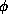whereis the angle the trajectory makes with respect to the forward tangent immediately after impact (Fig. 5). If we denote f as the mapping that transforms the coordinates (sn,zn) just after the nth collision to the coordinates (sn+1,zn+1) just after the (n+1)th collision [i.e., f: (sn,zn)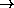(sn+1,zn+1)], then it has been shown that f is area preserving in the (s,z) coordinate representation [Birkhoff, 1927; Berry, 1981]. The map is undefined once the trajectory leaves the scattering region.

In this coordinate system, the three orbits that bounces back and forth between the disks indefinitely as shown in Fig. 5 are fixed points of the map located at: (0,0), (1/3,0), and (2/3,0). Using the same scheme as in Sec. 3, we now proceed to generate the basin picture for this new ``phase space''. The basin picture for the surface of section is shown in Fig. 6. The apparent basin boundaries formed by the lines s=0, s=1/3, and s=2/3 are artifacts of the way we constructed the surface of section. Initial conditions near these vertical lines experience discontinuous changes. For example, an initial condition on disk 1 near s=1/3 suddenly jumps to disk 2 as it crosses the line s=1/3 from s < 1/3 to s > 1/3. Henceforth, when we discuss boundary points in the surface of section, we are referring to all boundary points in Fig. 6 except the apparent boundary points lying on the lines s=0, s=1/3, and s=2/3. In addition, if we can show that these ``physical'' boundary points have the Wada property, then every boundary point in our ``physical'' surface of section plot of Fig. 3 also possess the Wada property. Note that the forward iterates of all physical boundary points remain in the scattering region forever. This follows, since, if the forward orbit of a point leaves through one of the exits in a finite number of iterates, then, by continuity (which does not apply at the map discontinuities, s = 0, 1/3, 2/3), that point necessarily has a neighborhood in which every point leaves through that exit. Thus a point cannot be a boundary point if its forward orbit does not remain in the scattering region forever.

The dynamics of the unstable invariant set in the three-disk model plays a crucial role in the formation of Wada basin boundaries, especially the stable and unstable manifolds of the invariant set. If f denotes the surface of section map, then the stable manifold of a point y in the invariant set is the set of points {x} whose forward iterates satisfy |fn(x) - fn(y)|0 as n. Thus, stable manifolds exist only for points y whose forward iterates remain in the scattering region for all time. Similarly, the unstable manifold of a point y in the invariant set is the set of points {w} whose backward iterates satisfy |f-n(w) - f-n(y)| as nwhere we assume that the backward orbit from y remains in the scattering region for all time. Since forward iterates of the boundary remain in the scattering region forever, the boundary set and the stable manifold of the invariant set are the same.

We implement the ``sprinkler algorithm'' used in Kantz & Grassberger  and Hsu et al.  to numerically approximate the unstable invariant set embedded in phase space along with its stable and unstable manifolds. Specifically, the phase space was partitioned into a fine grid of points, and each grid point was iterated t times. For sufficiently large t and a sufficiently fine grid, trajectories that remain in the scattering region after t iterates can be used to generate approximations to the relevant measures. In particular, the initial conditions for these trajectories approximate the stable manifold of the invariant set; the t-th iterates of the initial conditions approximate the unstable manifold of the invariant set; and the (=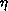t) iterates (0 << 1) approximate the unstable invariant set. Fig. 7(a) shows a picture of the invariant set, while Figs. 7(b) and (c) correspond to the stable and unstable manifolds of the invariant set respectively. There is an apparent symmetry when we compare the pictures of the stable and unstable manifolds. This is due to time-reversal symmetry, that is, the unstable manifold can be obtained from the stable manifold by simply reversing the direction of the trajectories. This corresponds to changing the angle fromto-or simply (s,z)(s,-z) since cos(-) = -cos. Figs. 7 show that the computed invariant set (Figs. 7(a)) is the intersection of the computed stable and unstable manifolds (Figs. 7(b) and 7(c)). (This is because orbits on the invariant chaotic set stay in the scattering region for all positive and negative time.) It is clear from Figs. 7 that, when the computed stable and unstable manifold cross, they do so at angles bounded well away from zero (i.e., they cross transversely). Thus we can conclude that the invariant chaotic set is hyperbolic. We also note that stable and unstable manifold segments terminate on the vertical lines s = 0, 1/3, 2/3.

We now show that all boundary points (not counting the lines s = 0, 1/3, 2/3) are Wada. Consider a boundary point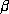. Since boundary points never exit, the orbit fromremains in the scattering region forever. It thus approaches the chaotic invariant set. Now consider a small disk D() of radiuscentered at. We want to show that, no matter how smallis, the disk D() contains pieces of all three basins. Consider the horizontal line segment L() of radiusthroughcontained in D() of radius. Since from Fig. 7(b), the slope dz/ds of the stable manifold of the invariant set is negative, we have that L() is transverse to the stable manifold of the invariant set. Thus, if we iterateand L() forward, the orbit from L() approaches the invariant set, and due to the hyperbolicity of the invariant set, the image of L() expands exponentially aligning itself with the unstable manifold of the invariant set (which from Fig. 7(c) has positive slope, dz/ds > 0). From inspection of Figs. 6 and 7 or of the picture on the cover of this issue which shows the chaotic set superposed on the basin structure, we conclude that we can choose an m large enough such that fm(L()) expands enough so that it manifestly crosses all three basins. [Note that finite precision computations (e.g., Fig. 6 of the basin structure are sufficient to draw this conclusion.] Now iterating this image fm(L()) backward m iterates to L(), we conclude that L() crosses all three basins. Hence D() contains points in all three basins, andis a Wada point. Sincecan be any point on the boundary, the entire basin boundary is Wada.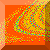Chaos at Maryland Home Page